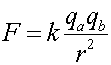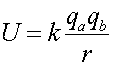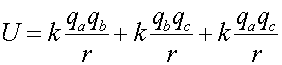Electric Potential Energy Just like in the gravitational case, the potential falls proportional to r-1. The form of the potential energy U looks the same as the that for the force F except for the power of r.Again, note that the potential energy is positive when the two charges have the same sign and negative otherwise. Note that the potential energy of a set of charges, qa,qb,...qz is the sum of the potential energies of the pairs. For instance, if there are 3 charges, qa,qb,qc, the net potential energy is: